### Home > GC > Chapter 1 > Lesson 1.2.4 > Problem1-85

1-85.

Copy the diagrams below on graph paper. Then find the result when each indicated transformation is performed.

1.    Reflect Figure A across line $l$.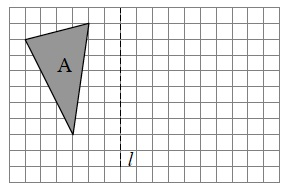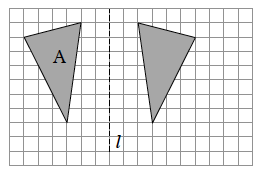1.   Rotate Figure B $90°$clockwise (${\circlearrowright}$) about point $P$.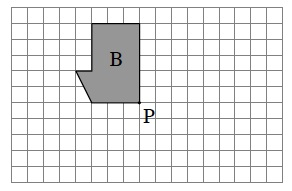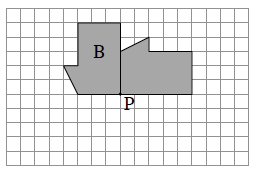1.   Reflect Figure C across line $m$.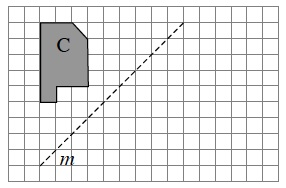See part (a) for help.

1.  Rotate Figure D $180°$about point $Q$.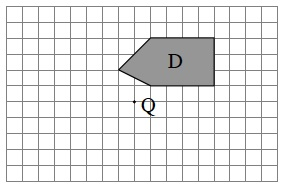See part (b) for help.